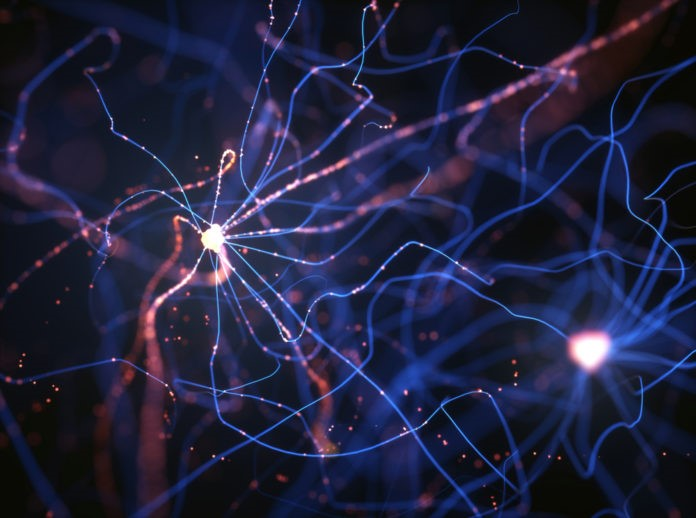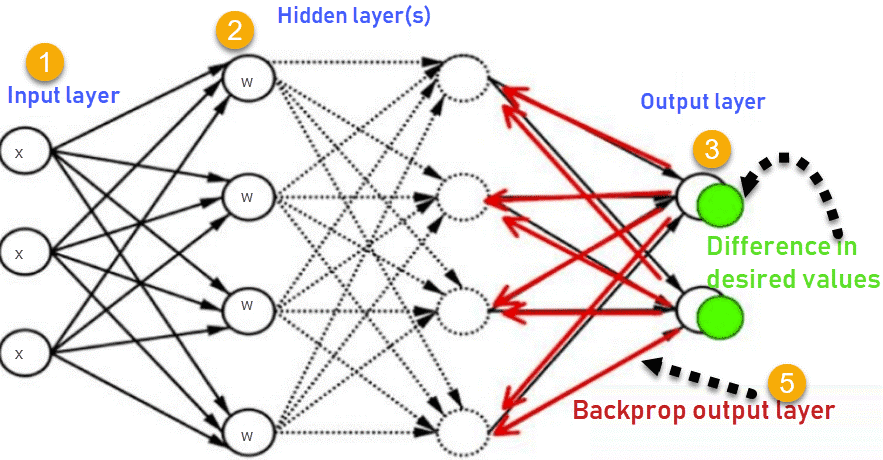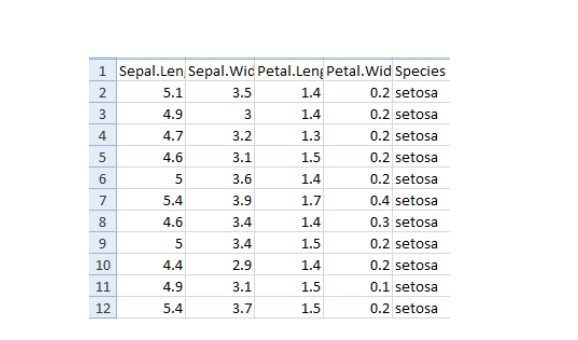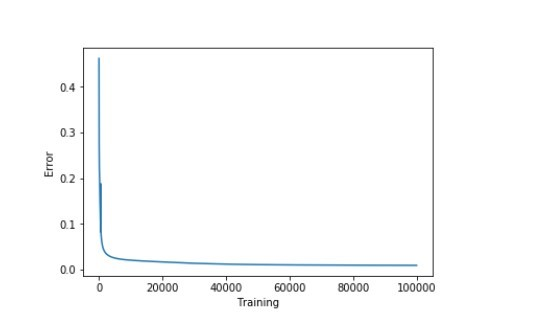# 反向传播算法详解1. 神经网络
2. 什么是反向传播？
3. 反向传播是如何工作的？
4. 损失函数
5. 为什么我们需要反向传播？
6. 前馈网络
7. 反向传播的类型
8. 案例研究

## 反向传播是如何工作的？（How does backpropagation work?）

1.输入层

2.隐藏层

3.输出层1.输入层接收x

2.使用权重w对输入进行建模

3.每个隐藏层计算输出，数据在输出层准备就绪

4.实际输出和期望输出之间的差异称为误差

5.返回隐藏层并调整权重，以便在以后的运行中减少此错误

## 损失函数（Loss function）

•反向传播快速、简单且易于实现

•没有要调整的参数

•不需要网络的先验知识，因此成为一种灵活的方法

•这种方法在大多数情况下都很有效

•模型不需要学习函数的特性

## 反向传播的类型（Types of backpropagation）

•静态反向传播（Static backpropagation）

•循环反向传播（Recurrent backpropagation）

1. 静态反向传播（Static backpropagation）

1. 循环反向传播（Recurrent backpropagation）

## 案例研究（Case Study）

``import numpy as np  import pandas as pd  import seaborn as sns  import matplotlib.pyplot as plt  from sklearn.model_selection import train_test_split``

``import warnings  warnings.simplefilter(action='ignore', category=FutureWarning)``

``iris = pd.read_csv("iris.csv")  iris.head()````iris. replace (, , inplace=True)``

1.对输出执行独热编码（one hot encoding）。

2.执行sigmoid函数

3.标准化特征

``def to_one_hot(Y):      n_col = np.amax(Y) + 1      binarized = np.zeros((len(Y), n_col))      for i in range(len(Y)):          binarized ] = 1.  return binarized``

``def sigmoid_func(x):      return 1/(1+np.exp(-x))  def sigmoid_derivative(x):      return sigmoid_func(x)*(1 – sigmoid_func(x))``

``def normalize (X, axis=-1, order=2):      l2 = np. atleast_1d (np.linalg.norm(X, order, axis))      l2 = 1  return X / np.expand_dims(l2, axis)``

``x = pd.DataFrame(iris, columns=columns)  x = normalize(x.as_matrix())    y = pd.DataFrame(iris, columns=columns)  y = y.as_matrix()  y = y.flatten()  y = to_one_hot(y)``

``#Split data to training and validation data（将数据拆分为训练和验证数据）  X_train, X_test, y_train, y_test = train_test_split(x, y, test_size=0.33)  #Weights  w0 = 2*np.random.random((4, 5)) - 1 #for input - 4 inputs, 3 outputs  w1 = 2*np.random.random((5, 3)) - 1 #for layer 1 - 5 inputs, 3 outputs  #learning rate  n = 0.1``

``errors = []``

``for i in range (100000):  #Feed forward network  layer0 = X_train  layer1 = sigmoid_func(np.dot(layer0, w0))  layer2 = sigmoid_func(np.dot(layer1, w1))  Back propagation using gradient descent  layer2_error = y_train - layer2  layer2_delta = layer2_error * sigmoid_derivative(layer2)  layer1_error = layer2_delta.dot (w1.T)  layer1_delta = layer1_error * sigmoid_derivative(layer1)  w1 += layer1.T.dot(layer2_delta) * n  w0 += layer0.T.dot(layer1_delta) * n  error = np.mean(np.abs(layer2_error))  errors.append(error)``

``accuracy_training = (1 - error) * 100``

``plt.plot(errors)  plt.xlabel('Training')  plt.ylabel('Error')  plt.show()````print ("Training Accuracy of the model " + str (round(accuracy_training,2)) + "%")``

Output: Training Accuracy of the model 99.04%

``#Validate  layer0 = X_test  layer1 = sigmoid_func(np.dot(layer0, w0))  layer2 = sigmoid_func(np.dot(layer1, w1))  layer2_error = y_test - layer2  error = np.mean(np.abs(layer2_error))  accuracy_validation = (1 - error) * 100  print ("Validation Accuracy of the model "+ str(round(accuracy_validation,2)) + "%")``

Output: Validation Accuracy 92.86%

•如果约束非常少，则系统可能不起作用

•过度训练，过多的约束会导致过程缓慢

•只关注少数方面会导致偏见

•输入数据是整体性能的关键

•有噪声的数据会导致不准确的结果

•基于矩阵的方法优于小批量方法（mini-batch）